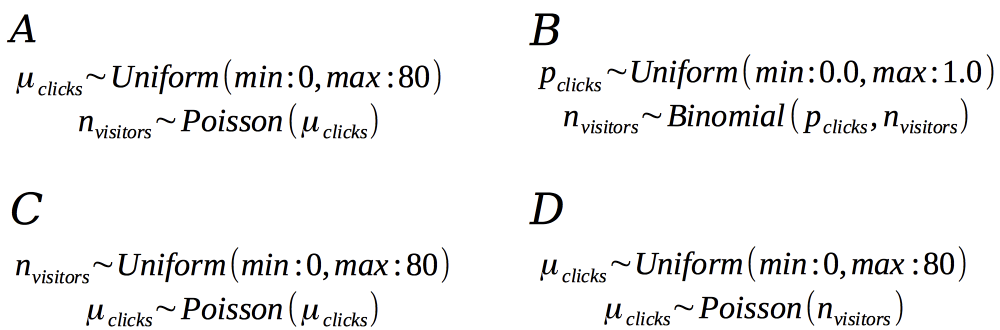# A Poisson model description

At the end of last chapter you tweaked the binomial model into a Poisson model using the following R code:

n_draws <- 100000
mean_clicks <- runif(n_draws, min = 0, max = 80)
n_visitors <- rpois(n = n_draws, mean_clicks)


Which of the following mathematical model descriptions best describe this model?